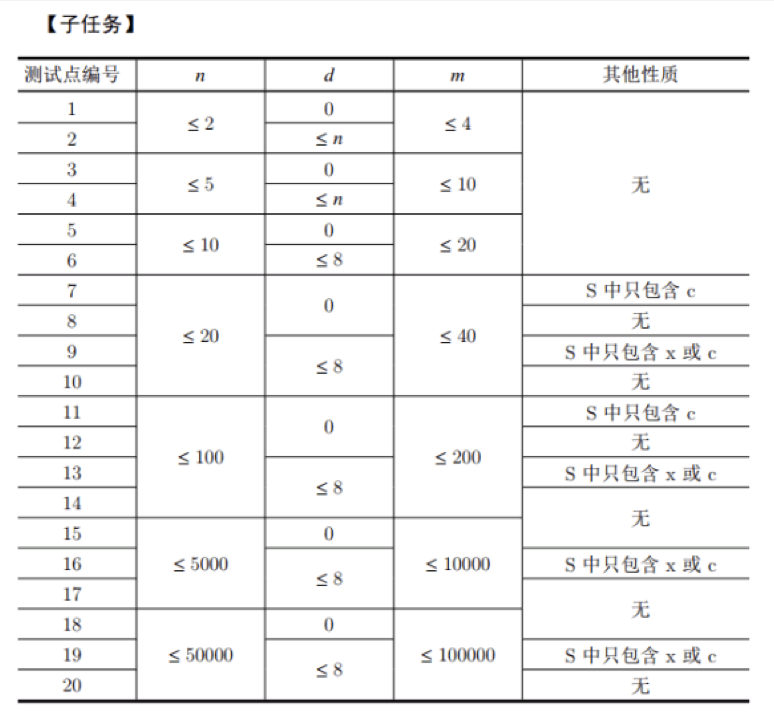# #2305. 「NOI2017」游戏

#### 题目描述

$n$ 场游戏的地图可以用一个小写字母组成的字符串描述。例如：$S=\texttt{xaabxcbc}$ 表示小L计划进行 $8$ 场游戏，其中第 $1$ 场和第 $5$ 场的地图类型是 $x$，适合所有赛车，第 $2$场和第 $3$ 场的地图是 $a$，不适合赛车 $A$，第 $4$ 场和第 $7$ 场的地图是 $b$，不适合赛车 $B$，第 $6$ 场和第 $8$ 场的地图是 $c$，不适合赛车 $C$

#### 输入格式

$n$, $d$, $S$ 的含义见题目描述，其中 $S$ 包含 $n$ 个字符，且其中恰好 $d$ 个为小写字母 $x$

#### 样例输入

3 1
xcc
1
1 A 2 B

#### 样例输出

ABA

$L$ 计划进行 $3$ 场游戏，其中第 $1$ 场的地图类型是 $x$，适合所有赛车，第 $2$ 场和第 $3$ 场的地图是 $c$，不适合赛车 $C$

$L$ 希望：若第 $1$ 场游戏使用赛车 $A$，则第 $2$ 场游戏使用赛车 $B$

#### 数据范围与提示